# How to Think Like a Computer Scientist: The PreTeXt Interactive Edition

## Exercises6.13Exercises

### 1.

Use the drawsquare function we wrote in this chapter in a program to draw the image shown below. Assume each side is 20 units. (Hint: notice that the turtle has already moved away from the ending point of the last square when the program ends.)Solution.
import turtle

def drawSquare(t, sz):
"""Make turtle t draw a square of with side sz."""
for i in range(4):
t.forward(sz)
t.left(90)

wn = turtle.Screen()       # Set up the window and its attributes
wn.bgcolor("lightgreen")

alex = turtle.Turtle()     # create alex
alex.color('hotpink')
alex.pensize(3)

for i in range(5):
drawSquare(alex, 20)   # Call the function to draw the square
alex.penup()
alex.forward(40)       # move alex to the starting position for the next square
alex.pendown()

wn.exitonclick()


### 2.

Write a program to draw this. Assume the innermost square is 20 units per side, and each successive square is 20 units bigger, per side, than the one inside it.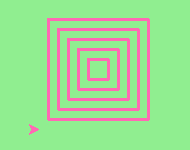### 3.

Write a non-fruitful function drawPoly(someturtle, somesides, somesize) which makes a turtle draw a regular polygon. When called with drawPoly(tess, 8, 50), it will draw a shape like this: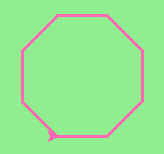Solution.
import turtle

def drawPoly(t, num_sides, side_length):
for i in range(num_sides):
t.forward(side_length)
t.left(360/num_sides)

wn = turtle.Screen()       # Set up the window and its attributes
wn.bgcolor("lightgreen")

tess = turtle.Turtle()
tess.color('hotpink')
tess.pensize(3)

drawPoly(tess, 8, 50)


### 4.

Draw this pretty pattern.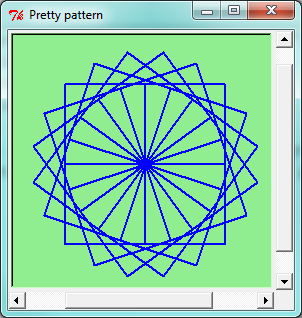### 5.

The two spirals in this picture differ only by the turn angle. Draw both.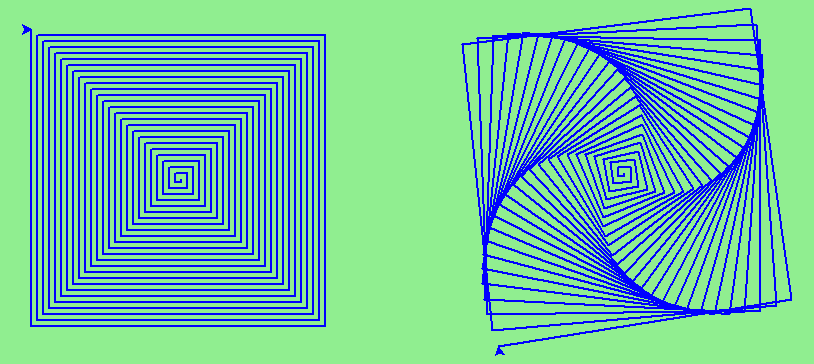Solution.
import turtle

def drawSpiral(t, angle):
''' takes a turtle, t, and an angle in degrees '''
length = 1
for i in range(84):
t.forward(length)
t.right(angle)
length = length + 2

wn = turtle.Screen()       # Set up the window and its attributes
wn.bgcolor("lightgreen")

guido = turtle.Turtle()    # create guido
guido.color('blue')

## draw the first spiral ##
# position guido
guido.penup()
guido.backward(110)
guido.pendown()

# draw the spiral using a 90 degree turn angle
drawSpiral(guido, 90)

## draw the second spiral ##
# position guido
guido.home()
guido.penup()
guido.forward(90)
guido.pendown()

drawSpiral(guido, 89)


### 6.

Write a non-fruitful function drawEquitriangle(someturtle, somesize) which calls drawPoly from the previous question to have its turtle draw a equilateral triangle.

### 7.

Write a fruitful function sumTo(n) that returns the sum of all integer numbers up to and including n. So sumTo(10) would be 1+2+3...+10 which would return the value 55. Use the equation (n * (n + 1)) / 2.
Solution.
from test import testEqual

def sumTo(n):
result = (n * (n + 1)) / 2
return result

# Now lets see how well this works
t = sumTo(0)
print("The sum from 1 to 0 is",t)
t = sumTo(10)
print("The sum from 1 to 10 is",t)
t = sumTo(5)
print("The sum from 1 to 5 is",t)


### 8.

Write a function areaOfCircle(r) which returns the area of a circle of radius r. Make sure you use the math module in your solution.

### 9.

Write a non-fruitful function to draw a five pointed star, where the length of each side is 100 units.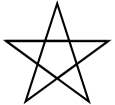Solution.
import turtle

def drawFivePointStar(t):
for i in range(5):
t.forward(100)
t.left(216)

wolfram = turtle.Turtle()
drawFivePointStar(wolfram)


### 10.

Extend your program above. Draw five stars, but between each, pick up the pen, move forward by 350 units, turn right by 144, put the pen down, and draw the next star. You’ll get something like this (note that you will need to move to the left before drawing your first star in order to fit everything in the window):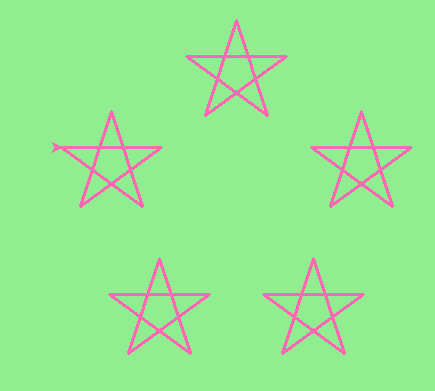What would it look like if you didn’t pick up the pen?

### 11.

Extend the star function to draw an n pointed star. (Hint: n must be an odd number greater or equal to 3).
Solution.
import turtle

def drawStar(t, n):
for i in range(n):
t.forward(100)
t.left(180 - 180/n)

stroustrup = turtle.Turtle()
drawStar(stroustrup, 7)


### 12.

Write a function called drawSprite that will draw a sprite. The function will need parameters for the turtle, the number of legs, and the length of the legs. Invoke the function to create a sprite with 15 legs of length 120.

### 13.

Rewrite the function sumTo(n) that returns the sum of all integer numbers up to and including n. This time use the accumulator pattern.
Solution.
def sumTo(n):
sum = 0
for i in range(1,n+1):
sum = sum + i
return sum

# Now lets see how well this works
t = sumTo(0)
print("The sum from 1 to 0 is",t)
t = sumTo(10)
print("The sum from 1 to 10 is",t)
t = sumTo(5)
print("The sum from 1 to 5 is",t)


### 14.

Write a function called mySqrt that will approximate the square root of a number, call it n, by using Newton’s algorithm. Newton’s approach is an iterative guessing algorithm where the initial guess is n/2 and each subsequent guess is computed using the formula: newguess = (1/2) * (oldguess + (n/oldguess)).

### 15.

Write a function called myPi that will return an approximation of PI (3.14159…). Use the Leibniz 1  approximation.
Solution.
def myPi(iters):
''' Calculate an approximation of PI using the Leibniz
approximation with iters number of iterations '''
pi = 0
sign = 1
denominator = 1
for i in range(iters):
pi = pi + (sign/denominator)
sign = sign * -1  # alternate positive and negative
denominator = denominator + 2

pi = pi * 4.0
return pi

pi_approx = myPi(10000)
print(pi_approx)


### 16.

Write a function called myPi that will return an approximation of PI (3.14159…). Use the Madhava 2  approximation.

### 17.

Write a function called fancySquare that will draw a square with fancy corners (sprites on the corners). You should implement and use the drawSprite function from above. For an even more interesting look, how about adding small triangles to the ends of the sprite legs.
Solution.
import turtle

def drawSprite(t, numlegs, leglength):
angle = 360/numlegs
for i in range(numlegs):
t.forward(leglength)
t.backward(leglength)
t.left(angle)

def drawFancySquare(t, sz, lgs, lgl):
for i in range(4):
t.forward(sz)
drawSprite(t, lgs, lgl)
t.left(90)

wn = turtle.Screen()
wn.bgcolor("lightgreen")

alex = turtle.Turtle()
drawFancySquare(alex, 100, 10, 15)

wn.exitonclick()


### 18.

There was a whole program in Section 6.11 to create a bar chart with specific data. Creating a bar chart is a useful idea in general. Write a non-fruitful function called barChart, that takes the numeric list of data as a parameter, and draws the bar chart. Write a full program calling this function. The current version of the drawBar function unfortuately draws the top of the bar through the bottom of the label. A nice elaboration is to make the label appear completely above the top line. To keep the spacing consistent you might pass an extra parameter to drawBar for the distance to move up. For the barChart function make that parameter be some small fraction of maxheight+border. The fill action makes this modification particularly tricky: You will want to move past the top of the bar and write before or after drawing and filling the bar.